## Digamma Function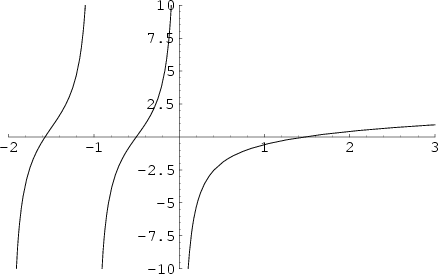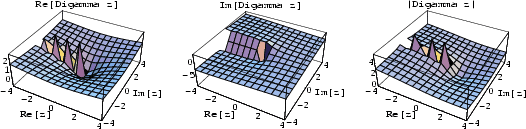Two notations are used for the digamma function. The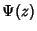digamma function is defined by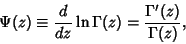(1)

where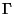is the Gamma Function, and is the function returned by the function PolyGamma[z] in Mathematica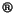(Wolfram Research, Champaign, IL). The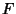digamma function is defined by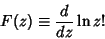(2)

and is equal to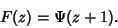(3)

From a series expansion of the Factorial function,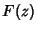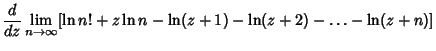(4)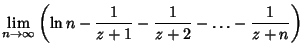(5)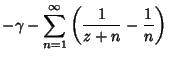(6)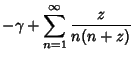(7)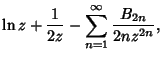(8)

where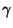is the Euler-Mascheroni Constant and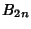are Bernoulli Numbers.

Theth Derivative ofis called the Polygamma Function and is denoted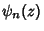. Since the digamma function is the zeroth derivative of(i.e., the function itself), it is also denoted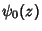.

The digamma function satisfies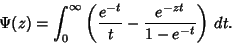(9)

For integral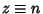,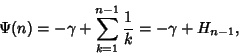(10)

whereis the Euler-Mascheroni Constant and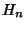is a Harmonic Number. Other identities include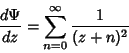(11)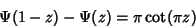(12)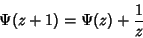(13)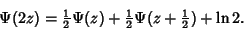(14)

Special values are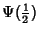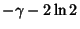(15)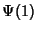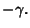(16)

At integral values,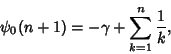(17)

and at half-integral values,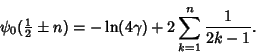(18)

At rational arguments,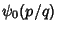is given by the explicit equation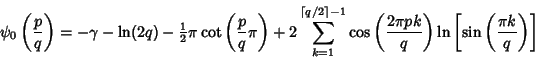(19)

for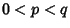(Knuth 1973). These give the special values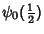(20)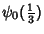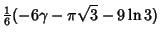(21)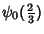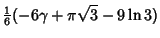(22)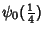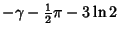(23)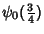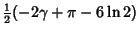(24)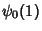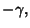(25)

whereis the Euler-Mascheroni Constant. Sums and differences of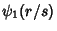for small integral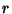and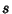can be expressed in terms of Catalan's Constant and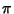.

References

Abramowitz, M. and Stegun, C. A. (Eds.). Psi (Digamma) Function.'' §6.3 in Handbook of Mathematical Functions with Formulas, Graphs, and Mathematical Tables, 9th printing. New York: Dover, pp. 258-259, 1972.

Arfken, G. Digamma and Polygamma Functions.'' §10.2 in Mathematical Methods for Physicists, 3rd ed. Orlando, FL: Academic Press, pp. 549-555, 1985.

Knuth, D. E. The Art of Computer Programming, Vol. 1: Fundamental Algorithms, 2nd ed. Reading, MA: Addison-Wesley, p. 94, 1973.

Spanier, J. and Oldham, K. B. The Digamma Function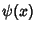.'' Ch. 44 in An Atlas of Functions. Washington, DC: Hemisphere, pp. 423-434, 1987.